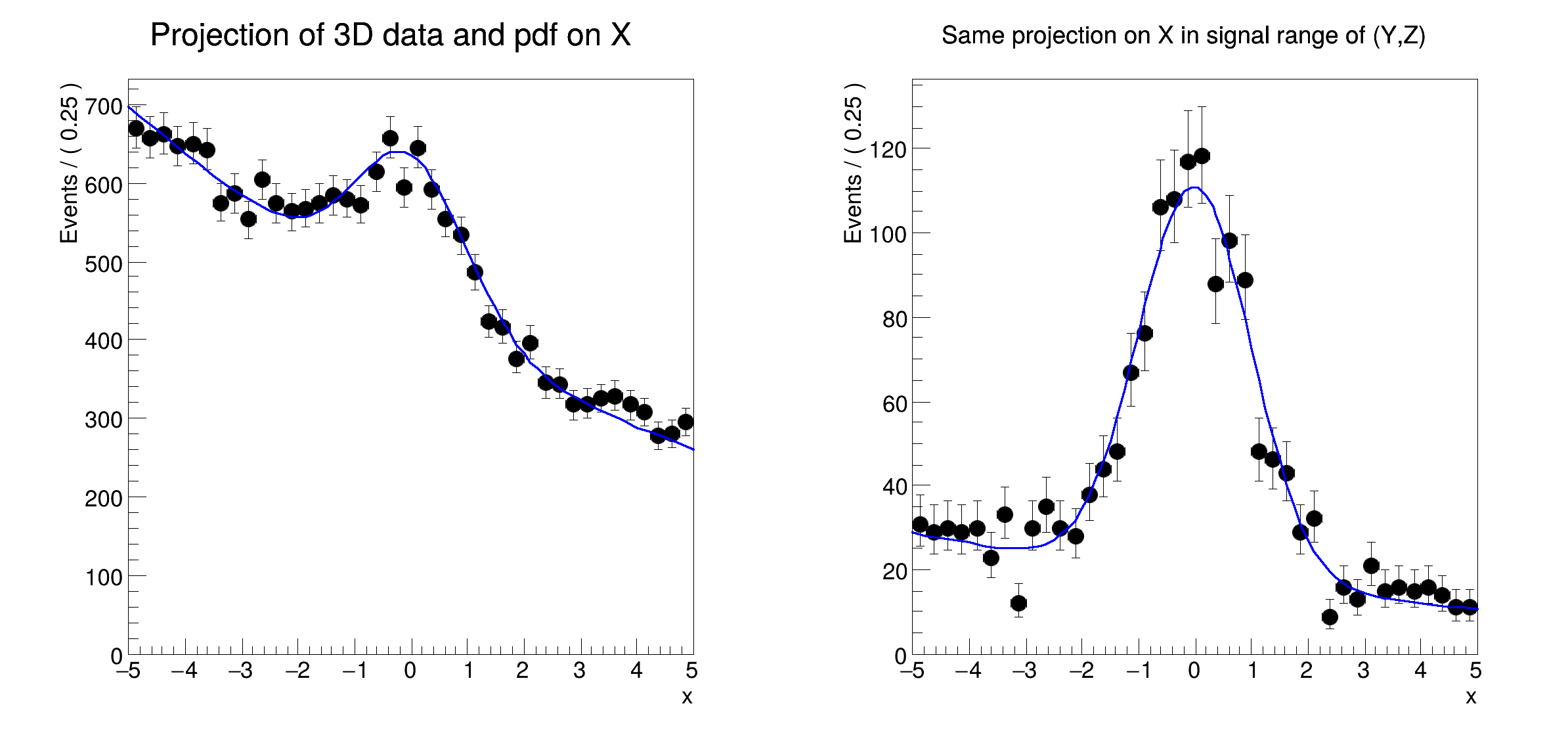ROOT   Reference Guide
Searching...
No Matches
rf311_rangeplot.C File Reference

## Detailed DescriptionMultidimensional models: projecting pdf and data ranges in continuous observables#include "RooRealVar.h"
#include "RooDataSet.h"
#include "RooGaussian.h"
#include "RooConstVar.h"
#include "RooProdPdf.h"
#include "RooPolynomial.h"
#include "TCanvas.h"
#include "TAxis.h"
#include "RooPlot.h"
using namespace RooFit;
{
// C r e a t e 3 D p d f a n d d a t a
// -------------------------------------------
// Create observables
RooRealVar x("x", "x", -5, 5);
RooRealVar y("y", "y", -5, 5);
RooRealVar z("z", "z", -5, 5);
// Create signal pdf gauss(x)*gauss(y)*gauss(z)
RooGaussian gx("gx", "gx", x, 0.0, 1.0);
RooGaussian gy("gy", "gy", y, 0.0, 1.0);
RooGaussian gz("gz", "gz", z, 0.0, 1.0);
RooProdPdf sig("sig", "sig", RooArgSet(gx, gy, gz));
// Create background pdf poly(x)*poly(y)*poly(z)
RooPolynomial px("px", "px", x, RooArgSet(-0.1, 0.004));
RooPolynomial py("py", "py", y, RooArgSet(0.1, -0.004));
RooPolynomial pz("pz", "pz", z);
RooProdPdf bkg("bkg", "bkg", RooArgSet(px, py, pz));
// Create composite pdf sig+bkg
RooRealVar fsig("fsig", "signal fraction", 0.1, 0., 1.);
RooAddPdf model("model", "model", RooArgList(sig, bkg), fsig);
std::unique_ptr<RooDataSet> data{model.generate({x, y, z}, 20000)};
// P r o j e c t p d f a n d d a t a o n x
// -------------------------------------------------
// Make plain projection of data and pdf on x observable
RooPlot *frame = x.frame(Title("Projection of 3D data and pdf on X"), Bins(40));
data->plotOn(frame);
model.plotOn(frame);
// P r o j e c t p d f a n d d a t a o n x i n s i g n a l r a n g e
// ----------------------------------------------------------------------------------
// Define signal region in y and z observables
y.setRange("sigRegion", -1, 1);
z.setRange("sigRegion", -1, 1);
// Make plot frame
RooPlot *frame2 = x.frame(Title("Same projection on X in signal range of (Y,Z)"), Bins(40));
// Plot subset of data in which all observables are inside "sigRegion"
// For observables that do not have an explicit "sigRegion" range defined (e.g. observable)
// an implicit definition is used that is identical to the full range (i.e. [-5,5] for x)
data->plotOn(frame2, CutRange("sigRegion"));
// Project model on x, integrating projected observables (y,z) only in "sigRegion"
model.plotOn(frame2, ProjectionRange("sigRegion"));
TCanvas *c = new TCanvas("rf311_rangeplot", "rf310_rangeplot", 800, 400);
c->Divide(2);
c->cd(1);
frame->GetYaxis()->SetTitleOffset(1.4);
frame->Draw();
c->cd(2);
frame2->GetYaxis()->SetTitleOffset(1.4);
frame2->Draw();
}
#define c(i)
Definition RSha256.hxx:101
Option_t Option_t TPoint TPoint const char GetTextMagnitude GetFillStyle GetLineColor GetLineWidth GetMarkerStyle GetTextAlign GetTextColor GetTextSize void data
RooAddPdf is an efficient implementation of a sum of PDFs of the form.
RooArgList is a container object that can hold multiple RooAbsArg objects.
Definition RooArgList.h:22
RooArgSet is a container object that can hold multiple RooAbsArg objects.
Definition RooArgSet.h:55
Plain Gaussian p.d.f.
Definition RooGaussian.h:24
A RooPlot is a plot frame and a container for graphics objects within that frame.
Definition RooPlot.h:43
static RooPlot * frame(const RooAbsRealLValue &var, double xmin, double xmax, Int_t nBins)
Create a new frame for a given variable in x.
Definition RooPlot.cxx:239
TAxis * GetYaxis() const
Definition RooPlot.cxx:1279
void Draw(Option_t *options=nullptr) override
Draw this plot and all of the elements it contains.
Definition RooPlot.cxx:652
RooPolynomial implements a polynomial p.d.f of the form.
RooProdPdf is an efficient implementation of a product of PDFs of the form.
Definition RooProdPdf.h:33
RooRealVar represents a variable that can be changed from the outside.
Definition RooRealVar.h:37
virtual void SetTitleOffset(Float_t offset=1)
Set distance between the axis and the axis title.
Definition TAttAxis.cxx:298
The Canvas class.
Definition TCanvas.h:23
RooCmdArg Bins(Int_t nbin)
RooCmdArg ProjectionRange(const char *rangeName)
RooCmdArg CutRange(const char *rangeName)
Double_t y[n]
Definition legend1.C:17
Double_t x[n]
Definition legend1.C:17
The namespace RooFit contains mostly switches that change the behaviour of functions of PDFs (or othe...
const char * Title
Definition TXMLSetup.cxx:68
[#1] INFO:Plotting -- RooAbsReal::plotOn(model) plot on x integrates over variables (y,z)
[#1] INFO:Eval -- RooRealVar::setRange(y) new range named 'sigRegion' created with bounds [-1,1]
[#1] INFO:Eval -- RooRealVar::setRange(z) new range named 'sigRegion' created with bounds [-1,1]
[#1] INFO:Plotting -- RooTreeData::plotOn: plotting 1692 events out of 20000 total events
[#1] INFO:Plotting -- RooAbsReal::plotOn(model) plot on x integrates over variables (y,z) in range sigRegion
Date
July 2008

Definition in file rf311_rangeplot.C.